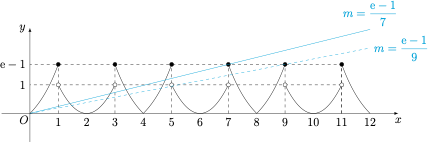# 每日一题整点个数

A．$\left(\dfrac{\mathrm{e}-1}{7}, \dfrac{\mathrm{e}-1}{5}\right]$

B．$\left[\dfrac{\mathrm{e}-1}{7}, \dfrac{\mathrm{e}-1}{5}\right]$

C．$\left(\dfrac{\mathrm{e}-1}{9}, \dfrac{\mathrm{e}-1}{7}\right]$

D．$\left[\dfrac{\mathrm{e}-1}{9}, \dfrac{\mathrm{e}-1}{7}\right]$